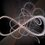# No L'Hospital's Rule

Can you prove that $\displaystyle \lim _{ x\rightarrow 1 }{ \frac { 1-{ x }^{ m } }{ 1-{ x }^{ n } } } =\frac { m }{ n }$ without using L'Hospital's rule for counting number m and n?

Hint: I did it by using the binomial theorem.
Bonus points if you can prove it for real number m and n, because my proof can't do that and I'd be interested to see it done.Note by Cole Wyeth
5 years, 7 months ago

This discussion board is a place to discuss our Daily Challenges and the math and science related to those challenges. Explanations are more than just a solution — they should explain the steps and thinking strategies that you used to obtain the solution. Comments should further the discussion of math and science.

When posting on Brilliant:

• Use the emojis to react to an explanation, whether you're congratulating a job well done , or just really confused .
• Ask specific questions about the challenge or the steps in somebody's explanation. Well-posed questions can add a lot to the discussion, but posting "I don't understand!" doesn't help anyone.
• Try to contribute something new to the discussion, whether it is an extension, generalization or other idea related to the challenge.

MarkdownAppears as
*italics* or _italics_ italics
**bold** or __bold__ bold
- bulleted- list
• bulleted
• list
1. numbered2. list
1. numbered
2. list
Note: you must add a full line of space before and after lists for them to show up correctly
paragraph 1paragraph 2

paragraph 1

paragraph 2

[example link](https://brilliant.org)example link
> This is a quote
This is a quote
    # I indented these lines
# 4 spaces, and now they show
# up as a code block.

print "hello world"
# I indented these lines
# 4 spaces, and now they show
# up as a code block.

print "hello world"
MathAppears as
Remember to wrap math in $$ ... $$ or $ ... $ to ensure proper formatting.
2 \times 3 $2 \times 3$
2^{34} $2^{34}$
a_{i-1} $a_{i-1}$
\frac{2}{3} $\frac{2}{3}$
\sqrt{2} $\sqrt{2}$
\sum_{i=1}^3 $\sum_{i=1}^3$
\sin \theta $\sin \theta$
\boxed{123} $\boxed{123}$

Sort by:

This solution goes up to rational numbers,

$\displaystyle \lim _{ x\rightarrow 1 }{ \frac { 1-{ x }^{ m } }{ 1-{ x }^{ n } } } =\lim _{ x\rightarrow 1 }{ \frac { \left( 1-{ x }^{ \frac { 1 }{ k } } \right) \left( 1+{ x }^{ \frac { 1 }{ k } }+{ x }^{ \frac { 2 }{ k } }+\cdots +{ x }^{ km } \right) }{ \left( 1-{ x }^{ \frac { 1 }{ k } } \right) \left( 1+{ x }^{ \frac { 1 }{ k } }+{ x }^{ \frac { 2 }{ k } }+\cdots +{ x }^{ kn } \right) } } for\quad any\quad k\\ =\lim _{ x\rightarrow 1 } \frac { \left( 1+{ x }^{ \frac { 1 }{ k } }+{ x }^{ \frac { 2 }{ k } }+\cdots +{ x }^{ km } \right) }{ \left( 1+{ x }^{ \frac { 1 }{ k } }+{ x }^{ \frac { 2 }{ k } }+\cdots +{ x }^{ kn } \right) } \\ =\frac { km }{ kn } \\ =\frac { m }{ n }$

- 4 years, 4 months ago

- 5 years, 7 months ago

Seems hardcore, but I'm a little confused by how you defined your variables at the beginning of the proof. Am I missing something?

- 5 years, 7 months ago

I am sorry, this is a bit confusing because I used "m" and "n" in the beginning also and the end also, but they have completely different roles

- 5 years, 7 months ago

well one can easily use taylor series, but a taylor series expansion is in a way equivalent to L'hospital rule when you really think about it, so would you accept that ?

$\lim _{ x-->1 }{ \frac { 1-(1+(x-1))^{ m } }{ 1-(1+(x-1))^{ n } } \quad \simeq \quad \frac { 1-(1+m(x-1)) }{ 1-(1+n(x-1)) } =\frac { m }{ n } }$

- 5 years, 7 months ago

I suppose most of math is pretty much equivalent- All this stuff is derived from limits, right? I think that counts.

- 5 years, 7 months ago

let S=limit as x tends to 1, (1-x^m)/(1-x^n) =limit as p tends to zero (1-e^pm)/(1-e^pn) ,where e^p=x.
applying expansion for e^pm and e^pn in numerator and denominator, S=lim as p tends to zero, (1-(1 + pm/1 + pm^2/2x1 + .........))/ (1-(1+ pn/1 + pn/2x1 +......)) , 1- 1 =0, in numerator and denominator , then taking ' m 'and 'n' outside from numerator and denominator , and substituting p=0 for rest of values, we get m/n. :) .pls correct me frnds, if there is any mistakes in my proof.

- 5 years, 7 months ago

@ Archit Boobna - " Since 1/kh will tend towards infinity..." You need grouping symbols around kh when it is written out horizontally, because of the Order of Operations:

1/(kh)

"We can easily show these (1+h)^2 = 1 + 2h (1+h)^4 = 1 + 4h (1+(1/2)h)^2 = 1 + h"

The above are not true. You cannot use equalities. You can use the equivalents of "leads to."

- 2 years, 1 month ago

Using the binomial theorem seems overkill. Writing it like this $\dfrac{1-x^m}{1-x} * \dfrac{1-x}{1-x^n}$ makes a much shorter solution apparent.

- 5 years, 7 months ago

How about the extension to real numbers?

It seems like L'Hopital needs to be used.

Staff - 5 years, 7 months ago